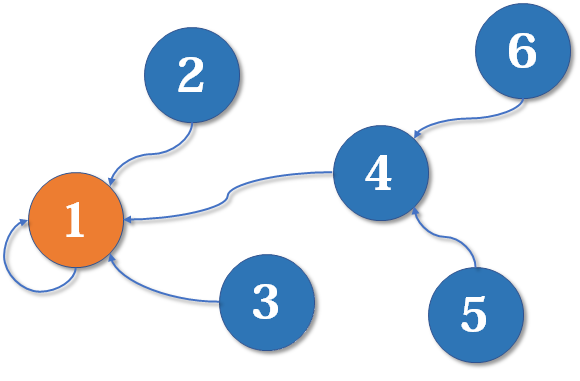4 7
2 1 2
1 1 2
2 1 2
1 3 4
2 1 4
1 2 3
2 1 4

N
Y
N
Y

# 二、具体实现

``````int f;
int find(int k){

return f[k]==k?k:f[k] = find(f[k]);
}
``````

1.如果找到的f数组内容就是本身查询的k值（自己就是自己的头子，相当于上述例子p=1），则直接返回k值
2.如果不是，就要去更深层挖掘，也就是我们写的递归程序，令f[k] = find(f[k])，一方面在寻找k的总头子（头子也可能有头子），一方面也直接把找到的值赋给了f[k]，我们管这叫路径压缩
（路径压缩优化后，并查集的时间复杂度已经比较低了，绝大多数不相交集合的合并查询问题都能够解决）

``````#include<bits/stdc++.h>
using namespace std;
int f;
int find(int k){

if(f[k]==k)return k;
else return f[k] = find(f[k]);

}
int main(){

int n,m;
cin >> n >> m;
for(int i=1;i<=n;i++)f[i] = i;
int type,a,b;
while(m--)
{

scanf("%d%d%d",&type,&a,&b);
if(type==1){

f[find(b)] = find(a);
}else
{

if(find(b)==find(a))
cout << "Y" << endl;
else
cout << "N" << endl;
}

}

return 0;
}
``````

# 三、拓展题

ZCMU-1435

Time Limit: 3 Sec Memory Limit: 128 MB
Submit: 592 Solved: 143

“M X Y” ：X国和Y国结盟 (如果X与Z结盟,Y与Z结盟,那么X与Y也自动结盟).

“S X” ：X国宣布退盟 (如果X与Z结盟,Y与Z结盟,Z退盟,那么X与Y还是联盟).

Input

Output

Sample Input

``````5 6
M 0 1
M 1 2
M 1 3
S 1
M 1 2
S 3
3 1
M 1 2
0 0
``````

Sample Output

``````Case #1: 3
Case #2: 2
``````

# 拓展题AC代码

``````#include<bits/stdc++.h>
using namespace std;
const int maxn = 2e6+5;
int f[maxn],ff[maxn],vis;
int find(int k){

if(f[k]==k)return k;
else return f[k] = find(f[k]);

}
int main(){

int m,n,ca = 1;
while(~scanf("%d%d",&n,&m))
{

int pos = n;
if(m==0&&n==0)break;
for(int i=0;i<maxn;i++)f[i] = ff[i] = i;
while(m--){

char str;
int a,b;
scanf("%s %d",str,&a);
if(str=='M'){

scanf("%d",&b);
int fa = find(ff[a]);
int fb = find(ff[b]);
if(fa!=fb)f[fa] = fb;
}else if(str=='S')
{

ff[a] = pos;
f[pos] = pos;
pos++;
}
}
set<int>s;
for(int i=0;i<n;i++)
s.insert(find(ff[i]));

printf("Case #%d: %d\n",ca++,s.size());

}
return 0;
}
``````

# 思路讲解`````` ff[a] = pos;
f[pos] = pos;
pos++;
//pos就是从n开始增大，因为题目数据范围是0 to n-1
``````

``````int fa = find(ff[a]);
int fb = find(ff[b]);
if(fa!=fb)f[fa] = fb;
``````